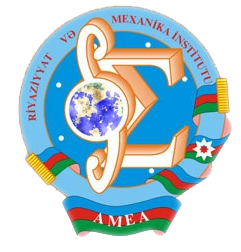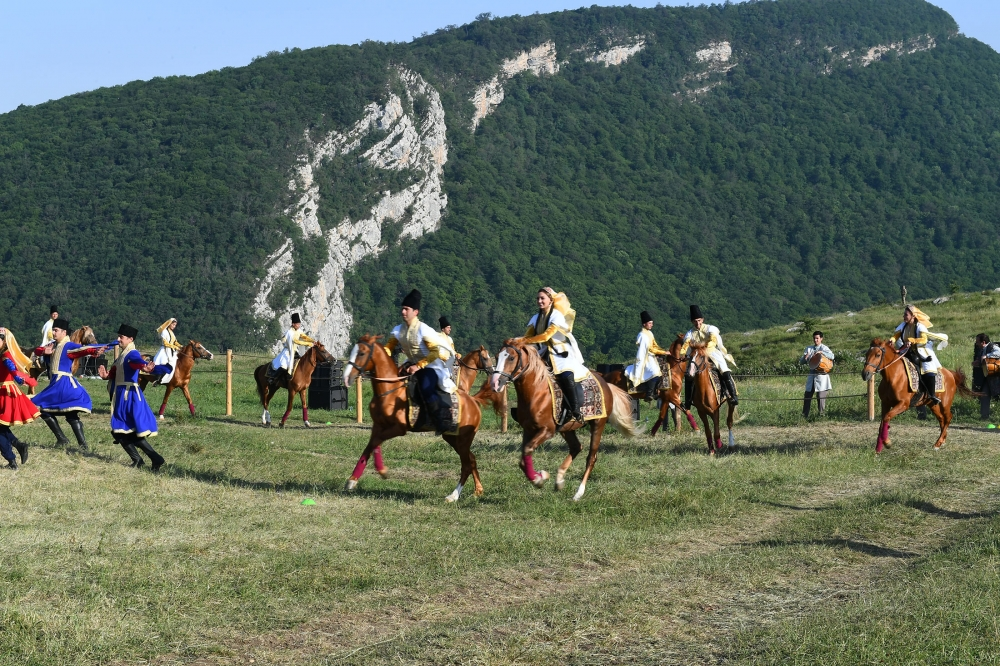Riyaziyyat və Mexanika İnstitutu

## Qarabağ xəbərləri### Şuşada Heydər Əliyev Fondunun təşkilatçılığı ilə “Musiqi irsi və Qarabağ atları Cıdır düzündə” adlı kompozisiya təqdim olunub## Transactions 2007/1/XXVII

 Year: 2007 Volume: 1 Number: XXVII MATHEMATICS Akhundov A.Ya. Abstract [PDF] Inverse problems for a system of parabolik equations[PDF] 3 Aliev A.R. Abstract [PDF] On the solvability of the equations containing in the main part the operators of the form-d3 dt3+A3 in the weighted space[PDF] 9 Aliev R.,Koca K.,Musayev B. Abstract [PDF] Approximate solutions of non-linear singular integral equations with Hilbert kernel [PDF] 17 Aliyev Z.S. Abstract [PDF] On basis properties of system of root functions of a fourth order spectral problem with spectral and physical parameters in boundary condition[PDF] 33 Aslanov G.I.,Gasimova G.N. Abstract [PDF] Investigation of resolvent of operator-differential equations on semi-axis[PDF] 45 Gasymov T.B.,Garayev T.Z. Abstract [PDF] On defective bases from the root elements of differential operators containing a spectral parameter in the boundary conditions [PDF] 51 Hajiyev V.H. Abstract [PDF] On generalized n-th order derivative functions square summable on Hilbert space with gauss measure[PDF] 55 Huseynov H.M.,Osmanova J.A. Abstract [PDF] On iost solution of Sturm-Liouville equation with discontinuity conditions[PDF] 63 Iskenderov B.A.,Mamedov J.Yu. Abstract [PDF] Behavior of the solution of the Cauchy problem for Barenblatt-Zheltov-Kochina type equation at great values of time [PDF] 71 Iskenderova M.B. Abstract [PDF] To the problem on multiple summability of expansions in eigen functions of irregular boundary value problems of fourth order[PDF] 79 Mamedov O.M. Abstract [PDF] Some commutator properties of a variety with a weak difference term[PDF] 89 Mekhtiev A.A. Abstract [PDF] Optimal control problem for bar oscillations equation [PDF] 95 Salimova G.A. Abstract [PDF] Solution of a boundary value problem for a partial differential equation or fourth order with not self-adjoint boundary conditions[PDF] 105 Shahbazov A.I.,Imanquliyev R.A. Abstract [PDF] Compact weighted composition operators on the space of holomorphic functions[PDF] 123 Suleymanov N.A. Abstract [PDF] On the existence of an absorbing set for semi-linear pseudohyperbolic equations of higher order [PDF] 129 Tagiyev.R.K. Abstract [PDF] The problems of optimal control by parabolic equations coefficients[PDF] 135 MECHANICS Aliyev A.A.,Shamiev F.H. Abstract [PDF] The carrying capacity of circular plates made of composite material,lying on incompressible fluid[PDF] 147 Mekhtiyev M.F. Abstract [PDF] Non-axially-symmetric problems of elasticity theory for transversally isotropic hollow sphere[PDF] 155 Najafov M.A. Abstract [PDF] Vibration and stability of a conic shell flitter in supersonic gas flow[PDF] 165 Ramazanov T.K.,Yusifova Sh.Ya. Abstract [PDF] Unsteady motion of the liquid in branching pipes [PDF] 173 APPLIED PROBLEMS OF MATHEMATICS AND MECHANICS Akhmedov N.K Abstract [PDF] Analysis of elastic waves propagation in radially laminated cylindrical waveguide[PDF] 181 Ibrahimov V.R.,Imanova M.N. Abstract [PDF] On a new method of solution to Volterra integral equation [PDF] 197 Imranov F.B. Abstract [PDF] On the convergence of a difference scheme approximating non-stationary problem of viscous incompressible liquid motion in closed domain in the presence of temperature distributions[PDF] 205 Mehdiyeva G.Yu. Abstract [PDF] On gradient methods in optimal control problems for nonlocal processes[PDF] 213
Azərbaycanda COVID-19 ilə bağlı statistika
• Virusa yoluxan

598503

• Sağalan

567385

• Yeni yoluxan

0

• Aktiv xəstə

23114

• Ölüm halı

8004

• Test edilib

5,621,021